Next: Grid-steered Molecular Dynamics Up: User-Defined Forces in NAMD Previous: TclForces

Subsections

TclBC

In a NAMD configuration file, one sets up the simulation parameters, such as the temperature, pressure, or a uniform electric field, for the molecular system as a whole. In many cases, though, you will need to impose boundary conditions, apply a non-uniform electric field, or selectively apply forces to a group of atoms of the given type or located in a particular area. For this purpose, you can use a tclBC (which stands for Tcl Boundary Conditions) script. A tclBC script is written in the Tcl programming language, a rudimentary knowledges of which would be enough for most tasks. The script will be interpreted line-by-line, therefore slowing down the simulation, sometimes considerably. For this reason you will want to keep your scripts simple and efficient.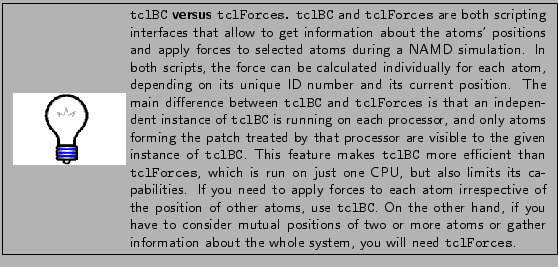The way the coordinates of atoms are wrapped around periodic boundaries in a tclBC script is controlled using the command wrapmode, which that may have one of the following arguments:

• patch is the default mode: the atom's coordinates are considered as the position in NAMD's internal patch data structure. This is in general different from an atom's coordinates relative to the global origin, so patch mode should not be used unless you know what you are doing.

• In mode input, the atom's coordinates correspond to its position in the input files of the simulation.

• In mode cell, the atom's equivalent position in the unit cell centered on the cellOrigin is used.

• In mode nearest, the atom's equivalent position nearest to the cellOrigin is used.

For most purposes, mode cell is a good choice.

Example 1: Making a Bubble

Imagine that you have a water box, and you want to create a spherical bubble of vacuum. You can do that by applying forces to every atom found inside this sphere to push it out. To avoid instability, you would want to start with a small bubble and then increase it until the desired size is reached. The rate of increasing the size of the bubble is up to you. If this rate is too large, some atoms will be pushed too hard and therefore move too fast, causing the simulation to crush. Fast-moving atoms may also break the structure of a double-stranded DNA or another molecular complex held together by relatively weak non-bonded interactions (van der Waals and electrostatic forces). Even if the simulation remains stable, you should watch the changes in temperature and pressure to make sure that the energy influx, which is equal to the work done by the force you are applying, has enough time to dissipate.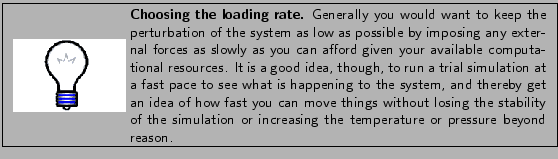1
Open the file tclBCfiles/BUBBLE/eq04.bubble.namd in a text editor. Scroll down to the end of the file to the line tclBC on.

You should find the following code:

tclBC on
tclBCScript {
set bubbleCenter    "0.0 0.0 0.0"
set tclBCScript     bubble.tcl
source $tclBCScript } tclBCArgs {0. 15. 0.01 5.} Since a tclBC script is called from NAMD, it is referenced NAMD configuration file, which is also a good place to set the script parameters. The NAMD command tclBC on turns the TclBC interface on. In our example, tclBCScript {...} contains the initialization of a key variable and a reference to the file that contains the script itself: source$tclBCScript. If the script is short, it can be placed entirely within the body of the command tclBCScript in the NAMD configuration file. Finally, the command tclBCArgs is used to pass a list of variables to the main TclBC procedure calcforces (we will talk about it very soon). In this case, the four arguments found in curly brackets have the meaning: make a bubble starting from radius 0 Å and increase it to 15 Å at a rate of 0.01 Å per simulation step, by applying forces of 5 kcal/molÅ.''

2
Look through the script tclBCfiles/BUBBLE/bubble.tcl.

bubble.tcl contains the following code:

# Two first agruments of calcforces are automatically forwarded
# to it by NAMD. The other 4 arguments match the list of 4 values
# from command tclBCArgs.

proc calcforces {step unique Rstart Rtarget Rrate K} {

global bubbleCenter ;# defined in tclBCScript{ ... }

# increase R, starting from $Rstart, by$Rrate at each step,
# until it reaches $Rtarget; then keep it constant set R [expr$Rstart + $Rrate *$step]
if { $R >$Rtarget } { set R $Rtarget } # let only the main processor print the output if {$unique } {
print "step $step, bubble radius =$R"
}

# get the components of the bubble center vector

foreach { x0 y0 z0 } $bubbleCenter { break } # pick atoms of the given patch one by one while {[nextatom]} { set rvec [getcoord] ;# get the atom's coordinates # get the components of the vector foreach { x y z }$rvec { break }

# find the distance between the atom and the bubble center
# (long lines can be broken by a backslash and continued
# on the next line)

set rho [expr sqrt(($x-$x0)*($x-$x0) + ($y-$y0)*($y-$y0) + \
($z-$z0)*($z-$z0))]

# if the atom is inside the sphere, push it away radially

if { $rho <$R } {
set forceX [expr $K * ($x-$x0) /$rho]
set forceY [expr $K * ($y-$y0) /$rho]
set forceZ [expr $K * ($z-$z0) /$rho]
addforce "$forceX$forceY $forceZ" } } }In the code bubble.tcl, we use the unique flag to print something from within calcforces once at each step. Without if {$unique } clause, the print command would be executed by every processor.

The list of variables Rstart Rtarget Rrate K forwarded to calcforces matches the list of values tclBCArgs {0.0 10.0 0.01 5.0} of the NAMD configuration file. You can use more or fewer variables, or none at all, as long as the lengths of the two lists are the same. The automatic variable step and unique don't count, so calcforces will always have two more arguments than to tclBCArgs.

The key command in calcforces is nextatom, which tells NAMD to select the next atom of the given patch. nextatom returns a value which is nonzero if the next atom has been successfully selected, or zero if no more atoms are left to process. Thus, while {[nextatom]} {...} is a loop that will be repeated until all atoms of the current patch are processed.

Once an atom is selected by nextatom, a number of commands can be used to get its properties:

set atomid [getid]     ;# 1-based atom ID number
set coords [getcoord]  ;# 3-component coordinate vector (A)
set mass   [getmass]   ;# mass (atomic units)
set charge [getcharge] ;# charge (atomic units)

Using these parameters, you can decide what to do to that atom.

In the script bubble.tcl, we find the coordinates of the atom using the command foreach { x y z } $rvec { break }; this is a standard trick to get the components of a vector or a list in Tcl. Using the same method, we find the coordinates of the bubble center. Then we check whether the atom is inside the bubble of radius$R and center $bubbleCenter. If so ($rho < $R), we calculate the components of the force vector such that it is directed radially from the bubble's center. In this simple example, the absolute value of the force is always$K whenever the force is applied. Finally, addforce tells NAMD to actually push the given atom by adding the force vector $forceX$forceY $forceZ'' to the force that would act on that atom otherwise. 3 Change (cd) to the directory tclBCfiles/BUBBLE. 4 Run a NAMD simulation by typing namd2 eq04.bubble.namd > eq04.bubble.log (for standard installations of NAMD.) 5 Open file eq04.bubble.log, and find a line that reports the speed of computation, e.g. TIMING: 3000 CPU: 1340.82, 0.4363/step. The speed of computation is given in seconds per step, one step being 1 fs in this case. Write these numbers down, as you will need to compare them to the speed of an optimized script. 6 If you are unable to run the simulation, use the sample output file ../BUBBLE_OUTPUT_EXAMPLE/eq04.bubble.log. 7 Open the simulation trajectory in VMD. Use the sample trajectory file ../BUBBLE_OUTPUT_EXAMPLE/eq04.bubble.dcd if necessary. 8 In main VMD window, click Graphics, then Representations. In Graphical Representations window, type x>0 in Selected Atoms text box, press Enter. Below this box, click Trajectory, select Update Selections Every Frame. Rotate the image if necessary using mouse. 9 In the main VMD window, click Display, then check Depth Cueing box. Click Display again, click Display Settings. Set Cue Mode to Linear. Set Cue Start 2.00, Cue End 3.00, vary them to see the effect of this parameters on the system's view. 10 Run the trajectory animation, and watch the formation of the bubble. Sample snapshots of the simulation trajectory are shown in Fig. 5. The directory tclBCmovies contains MPEG movies bubble*.mpg that show the bubble formation trajectory animation at 0.1 ps per frame.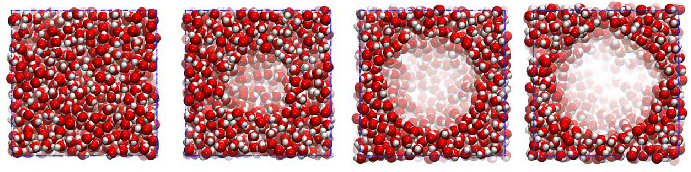Optimizing a TclBC script The above script bubble.tcl is far from being perfect. The same job can be done much more efficiently by decreasing the number of atoms processed at each step, as well as the number of arithmetic operations. Note that Tcl arithmetic is much slower than hard-coded C++ arithmetic of the NAMD. A more efficient script is given in file BUBBLE/bubbleFast.tcl.1 Take a look at the script BUBBLE/bubbleFast.tcl, also presented below: wrapmode cell # this line is moved above calcforces to avoid doing this # transformation more than once foreach { x0 y0 z0 }$bubbleCenter { break }

set TOL 3. ;# distance tolerance parameter

proc calcforces {step unique Rstart Rtarget Rrate K} {

global x0 y0 z0 TOL

set R [expr {$Rstart +$Rrate * $step}] if {$R > $Rtarget } { set R$Rtarget }

if { $unique } { print "step$step, bubble radius = $R" } # Atoms found at a distance larger than$TOL from the surface
# of the bubble, or $RTOL from the center of the bubble, will # be ignored (dropped) for the rest of a 100-step cycle. set RTOL [expr {$R + $TOL}] # restore the complete list of atoms to consider if {$step % 100 == 0 } { cleardrops }

while {[nextatom]} {

set rvec [getcoord]
foreach { x y z } $rvec { break } set rho [expr {sqrt(($x-$x0)*($x-$x0) + ($y-$y0)*($y-$y0) + \ ($z-$z0)*($z-$z0))}] # Atoms at distances 0 to$R from the bubble center are pushed,
# atoms father than $RTOL are dropped. Atoms between$R to $RTOL, # that is with a layer of thickness$TOL, are neither pushed nor
# dropped at this step, so that they will be considered again at
# the next step(s). They may come closer to the bubble and then
# they will have to be pushed.

if { $rho <$R } {

set forceX [expr {$K * ($x-$x0) /$rho}]
set forceY [expr {$K * ($y-$y0) /$rho}]
set forceZ [expr {$K * ($z-$z0) /$rho}]
addforce "$forceX$forceY $forceZ" } elseif {$rho > $RTOL } { dropatom ;# no longer consider this atom until "cleardrop" } } } Since most water molecules are far from the bubble, we don't need to check their coordinates every step. It's enough to do that once in a while (every 100 steps in this example). At all other times, only a small number of atoms that are still inside the bubble or within distance$TOL from it, and only these are selected by nextatom each step.

2
Open configuration file eq04.bubbleFast.namd, and make sure that it is calling the tclBC script bubbleFast.tcl. Run a NAMD simulation by typing

namd2 eq04.bubbleFast.namd > eq04.bubbleFast.log.

3
If you are unable to run the simulation, use the sample log file
../BUBBLE_OUTPUT_EXAMPLE/eq04.bubbleFast.log.

4
Look through file eq04.bubbleFast.log, find the word TIMING, and compare the speed to that found when using the script bubble.tcl. In our samples, the speeds obtained on a single CPU are 0.4363 s/step (8.0 ps/hr) and 0.2084 s/step (18.8 ps/hr) for the original and optimized script, respectively. Your numbers may differ.

5
Run a NAMD simulation using file eq04.bubbleFast.namd on a cluster. Make sure to direct the output to a new file. Repeat the above steps and find the computation speed. In our example
(../BUBBLE_OUTPUT_EXAMPLE/eq04.bubbleFast.4CPU.log), the speed on 4 CPUs increased to 0.0619 s/step (40.3 ps/hr).Example 2: Concentrating the ions in a solution

A more complex initialization of the TclBC script is necessary when it is supposed to process a selected group of atoms. Imagine that, for whatever reason, you want to gather all K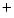and Clions within a sphere with the same center and radius as in the above example. For this purpose, we will push all ions toward the center of the sphere. The force applied will be constant for all ions beyond the sphere, and proportional to the distance between the ion and the sphere center for atoms inside the sphere.

1
Change to the directory tclBCfiles/IONS.

2
Open file eq04.concentrateIons.namd, scroll to the end, and look at the tclBC block.

As before, the tclBC script is set up in the NAMD configuration file:

tclBC on
tclBCScript {
set sphereCenter    "0.0  0.0  0.0"
set maxForce         5.0
set pdbSource       waterbox40-0.2M.pdb
set tclBCScript     concentrateIons.tcl
source $tclBCScript } tclBCArgs { } In this case, we don't explicitly pass any extra arguments to calcforces (the variables step and unique will still be passed implicitly). Instead, we set up global variables sphereCenter, sphereRadius, maxForce, and pdbSource. They will be made visible in calcforces using command global. To identify the ions, we will read the system's PDB file, locate the ions' entries, and make a list of their IDs. 3 Open the file waterbox40-0.2M.pdb in a text editor and find the lines containing text CLA (for chloride) or POT (for potassium). The ion entries in the PDB file for a large system may look like ATOM ***** CLA CLA 170 -36.100 -48.734 7.045 1.00 0.00 ION CL ... ATOM ***** POT POT 1 -0.574 -49.643 34.378 1.00 0.00 POT K In the above example, atom IDs for the ions are above 99999, and therefore not shown in the PDB file by a decimal. Thus, to find out the atom IDs of the ions, we will need to read the PDB file line by line while counting the atom entries. An entry for each atom must begin with four letters ATOM or HETA. In the PDB file above, each ion is identified by the residue name CLA or POT, which occupies positions 17-19 of the line. Note that a 4-letter residue name would occupy positions 17-20 of the line. To make the script more general, we will read symbols 17-20 and then trim them (remove spaces). 4 Open file concentrateIons.tcl, and look through it. The file concentrateIons.tcl begins with the following code: set ionList {} ;# a list of atom IDs of the ions set atomID 1 ;# in NAMD, atom IDs are 1-based numbers set inStream [open$pdbSource r]	;# opening the PDB file

# reading the PDB file line after line:
foreach line [split [read $inStream] \n] { # symbols 0-3 should be ATOM or HETA set string1 [string range$line 0 3]

# symbols 17-20 contain the residue name
set string2 [string range $line 17 20] set string2 [string trim$string2] ;# trimming the residue name

if { [string equal $string1 {ATOM}] || \ [string equal$string1 {HETA}] } {

# so it's a valid atom entry; let's see if this is an ion

if { [string equal $string2 {CLA}] || \ [string equal$string2 {POT}] } {

# yes it's an ion; append its index to the list
lappend ionList $atomID } # increase the atomID counter by 1 (default increment) incr atomID } } close$inStream

This part of the code, preceding proc calcforces, will be executed once by each processor. Identical instances of the list ionList of atom IDs for the ions will also be kept in memory at each processor.

Now we are ready to write the main part of the code:

wrapmode cell

# in this case the command tclBCArgs {} located in the NAMD config
# file doesn't pass any arguments to calcforces, therefore only
# step and unique will be listed below:

proc calcforces {step unique} {

# list all global variables set outside this procedure
# (except those we won't need, like pdbSource)

# find the components of the sphere's center

foreach { x0 y0 z0 } $sphereCenter { break } while {[nextatom]} { set atomid [getid] ;# get the ID of the current atom # check if this ID is listed in ionList; if it's found, # lsearch will return the position of the search pattern # in the list (a number >= 0), otherwise -1 is returned if { [lsearch$ionList $atomid] >= 0 } { set rvec [getcoord] ;# get the ion's coordinates foreach { x y z }$rvec { break }

# find the distance between the ion and the sphere's center

set rho [expr sqrt(($x-$x0)*($x-$x0) + ($y-$y0)*($y-$y0) + \
($z-$z0)*($z-$z0))]

# Apply same force $maxForce to each ion if it's outside the # sphere. The components of the force vector are chosen so # that the vector is directed toward the sphere's center, # and the vector's norm is$maxForce.

if { $rho >$sphereRadius } {

set forceX [expr -$maxForce * ($x-$x0) /$rho]
set forceY [expr -$maxForce * ($y-$y0) /$rho]
set forceZ [expr -$maxForce * ($z-$z0) /$rho]

} else {

# If the ion in already inside the sphere, scale the force
# by a factor of $rho/$sphereRadius, so that the force
# decreases from $maxForce to 0 as the ion approaches the # sphere's center: set forceX [expr -$maxForce * ($x-$x0) / $sphereRadius] set forceY [expr -$maxForce * ($y-$y0) / $sphereRadius] set forceZ [expr -$maxForce * ($z-$z0) / $sphereRadius] } # Finally, applying the force calculated above # to the current atom addforce "$forceX $forceY$forceZ"
}
}
}

5
Run a NAMD simulation with file eq04.concentrateIons.namd.

6
If you are unable to do that, find the example output files
eq04.concentrateIons.log and eq04.concentrateIons.dcd in the directory ../IONS_OUTPUT_EXAMPLE.

7
Open the log file eq04.concentrateIons.log, and find the speed of computation marked by keyword TIMING. In our example, it is 0.2038 s/step (17.2 ps/hr).

8
Load the simulation trajectory in VMD. Create two separate VDW representations for potassium (resname POT) and chloride (resname CLA) ions. For each of them, select Coloring Method: ColorID 4 (yellow) for Cland 12 (lime) for Kions.

9
Use the Molecule File Browser to load the same structure (waterbox40-0.2M.pdb and waterbox40-0.2M.psf files) again as a new molecule. Select this system in the Graphical Representations window, type "water" in the Selected Atoms box, and select coloring method ColorID 15 (iceblue) and drawing method MSMS with transparent material.

10
In Main window, double-click in column T of the molecule for which you created the ions' representations to make this molecule the top one. Run the animation and watch the ions gather in the center of the water box.

Sample snapshots of the ion concentration trajectory are shown in Fig. 6. The directory tclBCmovies contains MPEG movie ionConcentration.mpg that shows the process at 0.1 ps per frame.Example 3: Improving efficiency

The above code can be made both more computationally efficient, as well as more concise and elegant. In our case, we are pushing only the ions, therefore all other atoms can be safely dropped using the statement

if { [lsearch $ionList$atomid] == -1 } {

dropatom
continue

}

This way all non-ion atoms will be dropped at the first step and never selected again by nextatom until cleardrops is called, thus making the code more efficient. Some extra time could be saved if we didn't need to continue pushing the atoms that are already inside the sphere:

if { $rho <$sphereRadius } {
dropatom  ;# don't process this atom in the future
continue  ;# don't process this atom now, either
}

# time to time (every 100 steps in this example),
# let's restore the complete list of atoms
# to catch ions escaping from the sphere

if { $step % 100 == 0 } { cleardrops } Using the vector routines, instead of robust but lengthy (and therefore error-prone) code foreach { x0 y0 z0 }$sphereCenter { break }
foreach { x y z } $rvec { break } set rho [expr sqrt(($x-$x0)*($x-$x0) + ($y-$y0)*($y-$y0) + \ ($z-$z0)*($z-$z0))] ;# find the distance between two points # calculate the force components set forceX [expr -$maxForce * ($x-$x0) / $rho] set forceY [expr -$maxForce * ($y-$y0) / $rho] set forceZ [expr -$maxForce * ($z-$z0) / $rho] addforce "$forceX $forceY$forceZ" ;# apply the force

we could write briefly and clearly:

set relativePosition [vecsub $rvec$sphereCenter]
set rho              [veclength $relativePosition] set forceVector [vecscale$relativePosition \
[expr -$maxForce /$rho]]
addforce $forceVector or even more briefly (though maybe less clearly): set relativePosition [vecsub$rvec $sphereCenter] addforce [vecscale [vecscale$relativePosition \
[expr -$maxForce / [veclength$relativePosition]]]]

Another trick can simplify a tclBC script if you need to process all atoms of a certain type, as Kand Clin the above example. Since such atoms have unique masses, you could avoid reading the PDB file, and make the list of atoms by writing, e.g.:

while { [nextatom] } {
if { [getmass] > 35 && [getmass] < 40 } { ... }
}

Likewise, you could use if { [getcharge] } as a selection criterion. Just make sure that no other atoms could match the conditions of the selection.

1
Open the file concentrateIonsBrief.tcl, look through the code, partly explained above:

# we will use the following variable to calculate and print the
# average number of ions found outside the sphere at each step

set avgNumIons 0

set forceCoef [expr -$maxForce/$sphereRadius]

wrapmode cell

##################################################

proc calcforces {step unique} {

if { $step > 0 &&$step % 100 == 0 } {

# Calculate and print the average number ions found outside
# the sphere

set avgNumIons [expr $avgNumIons / 100.] print "Step$step, average number of ions outside \
the sphere: $avgNumIons" set avgNumIons 0 cleardrops } while {[nextatom]} { if { [getmass] < 35 || [getmass] > 40 } { dropatom ;# not a K+ or Cl-, forget about it continue } # vector between the ion and the sphere's center set relativePosition [vecsub [getcoord]$sphereCenter]
set rho              [veclength $relativePosition] if {$rho > $sphereRadius } { addforce [vecscale$relativePosition [expr -$maxForce/$rho]]
incr avgNumIons
} else {
dropatom ;# this ion is already inside the sphere
}
}
}

2
Note that in this code, the ions already inside the sphere are no longer pushed and are dropped. Can they escape from the sphere? 1

3
Run a NAMD simulation with file eq04.concentrateIonsBrief.namd that calls the tclBC script concentrateIonsBrief.tcl. If unable to do so, find the exemplary output concentrateIonsBrief.log and
concentrateIonsBrief.dcd in directory ../IONS_OUTPUT_EXAMPLE.

4
Open file concentrateIonsBrief.log, find the computation speed of the optimized script, compare it to the speed you found when using the original script. In our examples, the speeds are 0.1330 s/step (26.8 ps/hr) versus 0.2038 s/step (17.2 ps/hr).

5
In the file concentrateIonsBrief.log, find the lines that look like this: TCL: Step 100, average number of ions outside the sphere: 13.0. Plot the number of ions against simulation step. Hint: under a UNIX/Linux system, you can type grep outside eq04.concentrateIonsBrief.log | awk {print $3 " "$11}' > numIons.dat, and import the data from the file numIons.dat into xmgrace or another technical graphics program. Open the xmgrace file IONS_OUTPUT_EXAMPLE/numIons.agr to see a sample plot.

Example 4: Imposing a Shear Flow

As previously mentioned, the main feature of TclBC scripts is their ability to apply geometry-based forces. Using a TclBC script, it is possible to impose arbitrary fields of forces, thus imitating complex geometries and interactions while keeping the molecular system relatively small and simple. For example, a solution containing DNA in a microfluidic channel undergoes a shear flow, so that parallel layers of the liquid move with different velocities. We can simulate the shear flow by applying force to oxygen atoms of water molecules found within thin layers in XY planes at and around= zLo and= zHi, which variables will be set in the NAMD configuration file.

1
Change to the directory tclBCfiles/SHEAR, open file eq04.shear.namd, scroll to its end.

The following initialization is made in the NAMD configuration file:

tclBC on
tclBCScript {
set zLo          -15. ;# lower plane where forces are applied
set zHi           15. ;# top plane where forces are applied
set dz             3. ;# half-width of the layer
set TOL            6. ;# drop atoms that far from either layer
set force          5.
set pdbSource      dsDNA6_solv.pdb
set tclBCScript    shear.tcl
source $tclBCScript } tclBCArgs { } 2 Open the tclBC script shear.tcl, read it while paying attention to the comments (the same script is shown below). set atomList {} ;# a list of atom IDs of water oxygens set atomID 1 ;# in NAMD, atom IDs are 1-based numbers set inStream [open$pdbSource r]	;# opening the PDB file

# reading the PDB file line after line:
foreach line [split [read $inStream] \n] { # symbols 0-3 should be ATOM or HETA set string1 [string range$line 0 3]

# symbols 13-16 contain the atom's name
set string2 [string range $line 13 16] # trimming the atom's name set string2 [string trim$string2]

if { [string equal $string1 {ATOM}] || \ [string equal$string1 {HETA}] } {

# so it's a valid atom entry; let's see if
# this is a water's oxygen

if { [string equal $string2 {OH2}] } { # yes it is; append its index to the list lappend atomList$atomID
}
incr atomID
}
}
close $inStream puts "[llength$atomList] water oxygens atoms found"

set totalTorque 0.

wrapmode cell

####################################################

proc calcforces {step unique} {

global atomList shearStress zLo zHi dz TOL force

# the value of a global variable will be stored
# between calls to this procedure, while a local
# variable would be lost

global totalTorque

if { $step % 100 == 0 } { if {$totalTorque != 0. } {
print "Step $step, total torque applied:$totalTorque"
set totalTorque 0.
}
cleardrops
}

while {[nextatom]} {

# check if this atom should be considered at all

if { [lsearch $atomList [getid]] == -1 } { dropatom continue } # now check if it's within bounds zLo to zHi foreach { x y z } [getcoord] { break } ;# get coordinates if {$z >= [expr {$zLo-$dz}] && $z <= [expr {$zLo+$dz}] } { addforce "[expr {-$force}] 0.0 0.0"
set totalTorque [expr {$totalTorque -$force * $z}] } elseif {$z >= [expr {$zHi-$dz}] && \
$z <= [expr {$zHi+$dz}] } { addforce "$force 0.0 0.0"
set totalTorque [expr {$totalTorque +$force * $z}] } elseif { ($z >= [expr {$zLo-$TOL}] && \
$z <= [expr {$zLo+$TOL}] ) || \ ($z >= [expr {$zHi-$TOL}] && \
$z <= [expr {$zHi+\$TOL}] ) } {

continue ;# keep an eye on it, it may come closer next time

} else {

dropatom ;# this atom is too far, forget about it for now

}
}
}

3
Run a NAMD simulation with configuration file eq04.shear.namd that calls the tclBC script shear.tcl. Use the sample output from directory ../SHEAR_OUTPUT_EXAMPLE if necessary.

4
View the simulation trajectory in VMD. Use representation style Licorice for both DNA strands, coloring method ColorID 11 (purple) for segid ADNA and ColorID 7 (green) for segid BDNA. For selection water, use style Lines, ColorID 15 (iceblue).

5
Why were zLo and zHi not set to be the lower and upper boundaries of the system? 2

6
Open the output file eq04.shear.log, find a line with the total torque output. Plot the total torque versus simulation step. Use the hint from the previous section to extract the data. Open xmgrace file
SHEAR_OUTPUT_EXAMPLE/torque.agr to see a sample plot.

7
Run the same simulation on a cluster. How many times is the `total torque'' printed at each checkpoint? Can you modify the TclBC script to print a system-wide total just once each time? 3

Sample snapshots of the simulation trajectory are shown in Fig. 7. MPEG movies (found in directory tclBCmovies) shearSlow.small.mpg and
shearSlow.big.mpg show the trajectory animation at 0.1 ps per frame. At this slow framerate, the movement of water layers is clearly visible. In movies shearFast.small.mpg and shearFast.big.mpg, the frame rate is 1 ps per frame, which allows one to see the slow rotation and dissociation of DNA in the shear flow.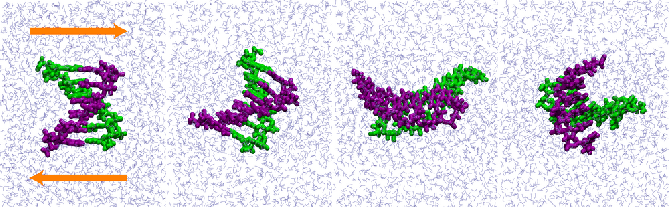Next: Grid-steered Molecular Dynamics Up: User-Defined Forces in NAMD Previous: TclForces
tutorial-l@ks.uiuc.edu